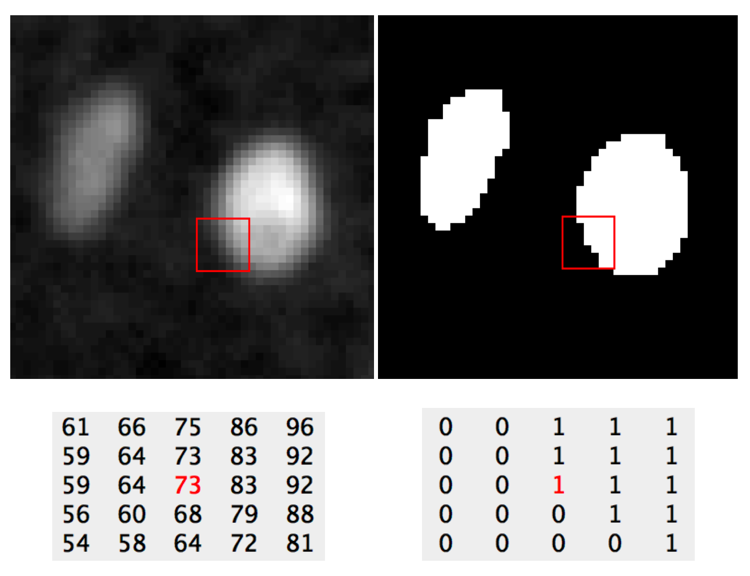## Prerequisites

Before starting this lesson, you should be familiar with:

## Learning Objectives

After completing this lesson, learners should be able to:
• Describe the relationship between an intensity image and a derived binary image

• Apply a threshold to segment an image into foreground and background regions

## Motivation

One strategy to detect objects or specific regions in images is to first distinguish so-called background pixels, which do not contain objects or interesting regions from foreground pixels, which mark the areas of interest. This process is called two class semantic segmentation and is often referred to as image binarization. The foreground regions can then be further processed, e.g. to detect objects or perform intensity measurements.

## Concept map

graph TD I("Image") --> T("Threshold") T --> BI("Binary image / Binary mask") BI --- BG("Background pixels (false, 0)") BI --- FG("Foreground pixels (true, 1, 255)")

## FigureImage before and after applying a threshold of 73 gray values.

## Activities

#### Threshold bright and dim cells

• Open xy_8bit__two_cells.tif
• Visualise the image and inspect its value range using a histogram
• Threshold the image to segment both cells
• Threshold the image to only segment the brighter cell

Show activity for:

## ImageJ GUI

• Find and apply a threshold
• Open xy_8bit__two_cells.tif
• Set binary options: [Process > Binary > Options ..]
• Black background
• Inspect pixel values to find a threshold separating fore- and background
• Hover over the image and observe the pixel values in ImageJ status bar
• Draw line profile and [Analyze > Plot Profile ] or [Ctrl-K]
• Inspect histogram using [Analyze > Histogram] or [Ctrl-H]
• If you do this for the whole image the background peak dominates the histogram
• Draw a ROI on the image such that there is 50/50 foreground and background
• Interactively find and apply a threshold
• [Image > Adjust > Threshold…] or [Ctrl-Shift-T]
• Dark Background
• Upper slider is lower threshold level, which is the value that you observed in the aforementioned step that would separate foreground and background
• Lower slide is the upper threshold level. This can be set to the maximum value of the bit depth (in this case 255)
• Press `Apply` to create a binary image
• Appreciate that the binary image only has two values: 0 and 255
• Repeat the operation so that only the brightest cell remains visible

## skimage napari

#### Spots and threshold interval

• Open image xy_8bit__PCNA.tif
• Find a threshold value so that there are 2 foreground nuclei
• Find a threshold value so that only the bright dots remain
• Find a threshold interval so that only the boundaries of the nuclei remain

Show activity for:

## ImageJ GUI

• [File > Open…] xy_8bit__PCNA.tif
• Use [Analyze > Plot Profile ]/[Ctrl-K] to identify possible threshold values
• Use [Image > Adjust > Threshold…] to create binary masks. Suggestion: Duplicate the image first , do not forget to press `Apply`

Example solutions:

1. `Lower threshold level` ~ 5
2. `Lower threshold level` ~ 44
3. `Lower threshold level` ~ 4 and `Upper threshold level` ~ 4-5

## skimage napari

#### Threshold based on noisemeasurement

Here we set the threshold value `t` such that it is higher than the background intensity plus some noise level.

• Open the image xy_8bit__two_cells.tif
• Measure the mean `m` and standard deviation `s` of the intensity in a backgroun region.
• Set the threshold as `t = m + N*s` choosing some `N` larger than 1; `N` effectively determines the statistical significance with which the values above `t` are foreground pixels.

Show activity for:

## ImageJ GUI

• Open xy_8bit__two_cells.tif
• Draw a squared ROI
• [Analyze > Measure] or [Ctrl-M}
• Eventually add `[x] Mean gray value` and `[x] Standard deviation` to your result table, [Results > Set Measurements]
• Note the value of `Mean` and `StdDev` and compute their sum
• Choose a multiple of this sum for the threshold
• Press `Apply` to create a binary image

## Assessment

### Fill in the blanks

• Pixels in a binary image can have maximally ___ different values.
• If the threshold is larger than the maximal pixel value in the intensity image, all pixels in the binary image have a value of ___.

## Solution

• Pixels in a binary image can have maximally 2 different values.
• If the threshold is larger than the maximal pixel value in the intensity image, all pixels in the binary image have a value of 0.

### True or False

• There is only one correct threshold value in order to convert an intensity image into a binary image.
• Binary images are always unsigned 8-bit where the foreground is 255.

## Solution

• There is only one correct threshold value in order to convert an intensity image into a binary image. False
• Binary images are always unsigned 8-bit where the foreground is 255. False

## Explanations

A common algorithm for binarization is thresholding. A threshold value `t` is chosen, either manually or automatically, and all pixels with intensities below `t` are set to 0, whereas pixels with intensities `>= t` are set to the value for the foreground. Depending on the software the foreground value can be different (e.g. 1 in MATLAB or 255 in ImageJ). At any pixel `(x,y)`:

`p_im(x,y) < t` `->` `p_bin(x,y) = 0`

`p_im(x,y) >= t` `->` `p_bin(x,y) = 1`

where, p_im and p_bin are the intensity and binary images respectively.

It is also possible to define an interval of threshold values, i.e. a lower and upper threshold value. Pixels with intensity values within this interval belong to the foreground and vice versa.

## Follow-up material

Recommended follow-up modules: